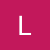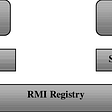Leetcode pattern : The Histogram pattern

I am going to name it as the histogram pattern as it was a histogram problem that first motivated me to understand this pattern.

The related problems on leetcode are :

84. Largest Rectangle in Histogram

85. Maximal Rectangle

Let’s go ahead with the first problem : Largest rectangle in a histogram.

As seen above, given a histogram with bars of varying length, how do you evaluate the maximum area covered by the largest rectangle in the histogram?

One way to solve the problem would be using a stack. Think about it : say your pointer is on block ‘i’ with a height 6, the next block (i+1) has a height of 2. By taking the ‘i+1’ th block into consideration the max area would reduce (it would be 2*2 now from 6 earlier (due to the ith block)). Whereas, if the ‘i+1’ th block had a height of say 7, then the max area would increase : it would be (6*2 = 12) now.

So, one way to solve the problem would be to keep on pushing blocks into the stack until you encounter one that has a smaller height. Then you evaluate the maxArea by peeking the top-most block of the stack as the one with the minimum height.

`public int largestRectangle(int[] height) {    Stack<Integer> stack = new Stack<>();    int current = 0, maxArea = 0;    while(current < height.length) {        if(stack.isEmpty() ||            height[stack.peek()] < height[current]) {           stack.push(current++);        } else {           int top = stack.peek();           stack.pop();           maxArea = Math.max(maxArea,            height[top] *            (stack.isEmpty() ? i : i - stack.peek() -1);         }}    while(!stack.isEmpty()) {           int top = stack.peek();           stack.pop();           maxArea = Math.max(maxArea,            height[top] *            (stack.isEmpty() ? i : i - stack.peek() -1);       }return maxArea;}`

Now, moving on to problem 85. Given a 2D binary matrix filled with 0’s and 1’s, find the largest rectangle containing only 1’s and return its area.

`Input:[  ["1","0","1","0","0"],  ["1","0","1","1","1"],  ["1","1","1","1","1"],  ["1","0","0","1","0"]]Output: 6from : Leetcode`

One way to look at the above problem is reducing it to a histogram. If a column has 1 already, then we add it to the previous column value.

--

--

--

## More from Sweta Barman

I’m a software engineer with a strong interest in algorithms. I love solving puzzles and love the aha moment of arriving at a solution after hours of struggle.

Love podcasts or audiobooks? Learn on the go with our new app.## Sweta Barman

I’m a software engineer with a strong interest in algorithms. I love solving puzzles and love the aha moment of arriving at a solution after hours of struggle.

## Quick and dirty guide to code reviews## Java RMI Basics (Remote method Invocation)